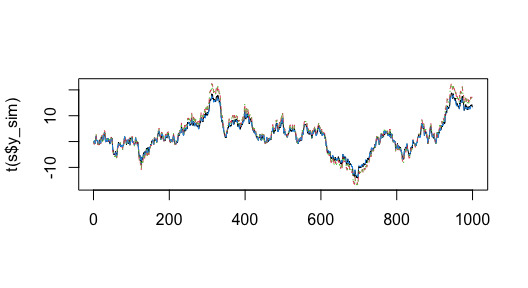Examples of fitting DFA models with lots of data

2021-09-28

In addition to fitting conventional DFA models with trends modeled as random walks (or ARMA processes), we can also construct models where underlying trends are treated as smooth trends (B-splines, P-splines, or Gaussian processes).

library(bayesdfa)
library(ggplot2)
library(dplyr)
library(rstan)
chains = 1
iter = 10

Data simulation

The sim_dfa function normally simulates loadings $$\sim N(0,1)$$, but here we will simulate time series that are more similar with loadings $$\sim N(1,0.1)$$

set.seed(1)
s = sim_dfa(num_trends = 1, num_years = 1000, num_ts = 4,